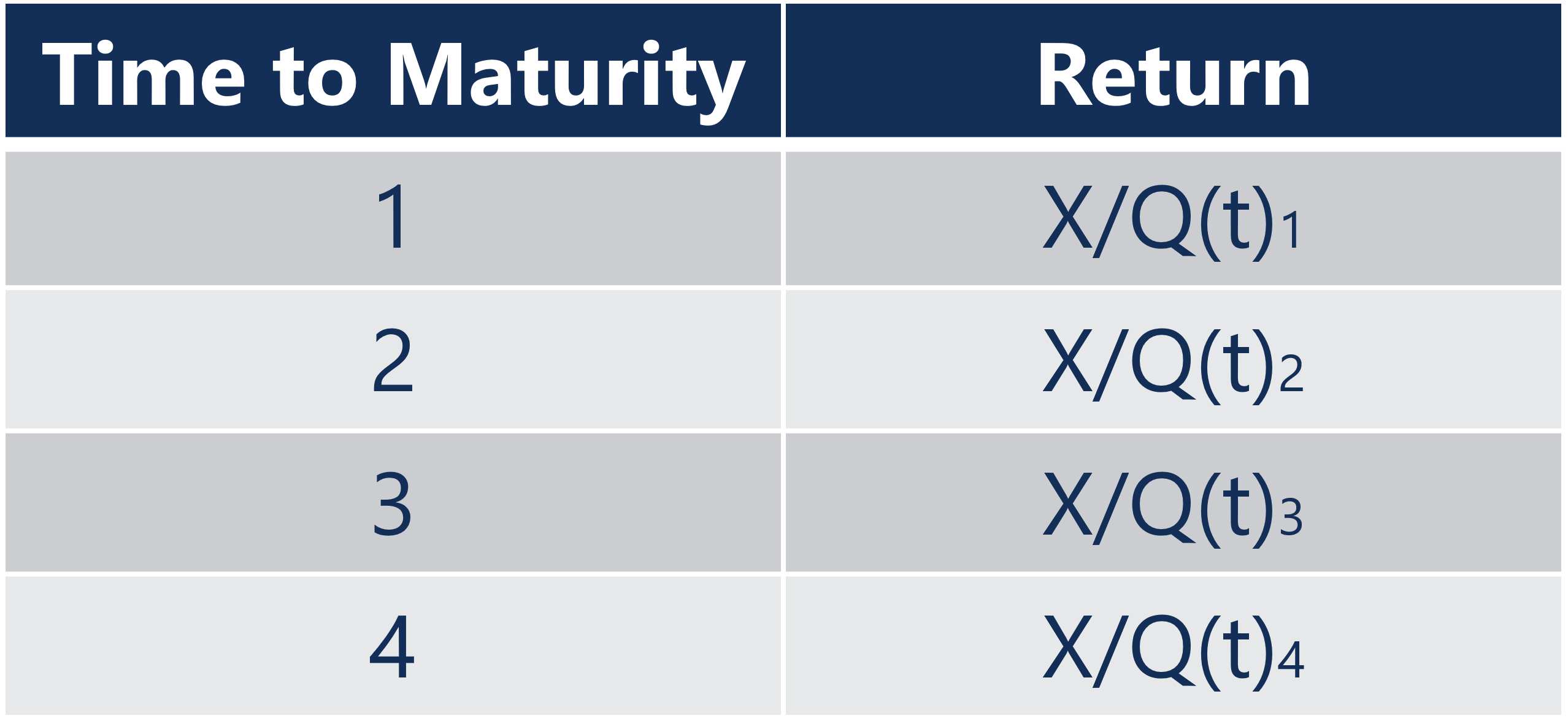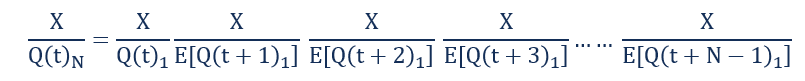# Forward Rates Models

Frameworks used predict the expected value of economic variables in the future

## What are Forward Rates Models?

Forward rates models are theoretical frameworks used to analyze and predict the expected value of economic variables in the future. Forward rates usually refer to either the forward interest rate or the forward exchange rate.### Expectations Hypothesis

Consider the following example: An N-year government bond costs Q(t)N in period t and pays an amount X in period t+N years. Therefore, the return on a 1-year bond is X/Q(t)1. The 1-year bond pays X in period t+1.Consider an investor who does the following:

1. Buys a 1-year bond now by paying Q(t)1
2. At the end of the year receives X
3. At the end of the year, spends all of X on buying 1-year bonds that will expire at the end of next year

The return on a sequence of two 1-year investments is X/Q(t)1 * X/Q(t+1)1.

If investors are risk neutral, then the return on a 2-year bond should be equal to the expected return on a sequence of two 1-year bonds. In general, the expected return on N 1-year bonds should be equal to the return on one N-year bond.The ‘E’ denoted around future bond prices in the denominator represents the expected values since investors don’t know the future bond prices.

### Forward Interest Rate

The expectations hypothesis can be used as a model to derive forward interest rates and exchange rates. The forward interest rate is the expected rate of interest offered by a security in the future. The forward interest rate can be inferred by analyzing the term structure of interest rates.

Consider the following example:

• A \$1 2-year zero coupon bond gives a return of 12% per year.
• A \$1 1-year zero coupon bond gives a return of 9% per year.

An investor investing in a \$1 2-year zero coupon bond will have \$1.2544 at the end of two years. An investor investing in a \$1 1-year zero coupon bond will have \$1.09 at the end of one year. If the investor proceeded to invest in a \$1 1-year zero coupon bond at the end of the first year, the return would depend on the future interest rate offered by a \$1 1-year zero coupon bond. The implied 1-year forward rate is that rate of interest that rules out the possibility of arbitrage. Since there is no possibility of arbitrage, the expectations hypothesis says that the product of the two 1-year rate should equal the 2-year rate. Therefore, the answer is 1.09(1 + rforward) = 1.2544, implying a 1-year forward rate of 15.08%.

Learn more about all the different types of fixed income securities with CFI’s Fixed Income Fundamentals Course!

### Forward Exchange Rate

The forward exchange rate between two currencies is the exchange rate between two currencies when the actual exchange takes place in the future. Consider the following example: The current USD/GBP exchange rate is £1 = \$1.2. It is known as the spot exchange rate or the exchange rate faced by a currency trader willing to deliver or take delivery of either USD or GBP right now.

The current 1-year risk-free rate of interest in the US is 5%. Therefore, \$1 deposited in a bank in the US earns interest worth \$0.05. The current 1-year risk-free rate of interest in the UK is 3%. Therefore, a £1 deposited in a bank in the UK earns an interest of £0.03.

Consider a currency trader in the USD/GBP market who initially has £1. At the end of one year, the trader can either have £1.03 or \$1.26. The efficient 1-year forward exchange rate is the exchange rate that rules out the possibility of arbitrage in the USD/GBP market.  Therefore, the 1-year forward USD/GBP exchange rate is £1 = \$1.22, which is higher than the spot rate. This forward rate neutralizes any possible arbitrage that an investor could have if they held USD – which has a higher interest rate – than GBP.

CFI is the official provider of the Financial Modeling and Valuation Analyst (FMVA)™ certification program, designed to transform anyone into a world-class financial analyst.

To keep learning and developing your knowledge of financial analysis, we highly recommend the additional resources below:

• Coupon Rate
• Interest Rate Swap
• Swap Rate Curve